# A Comprehensive Guide to Time Series Analysis in PythonThanks to the vast volumes of data being generated each day, it’s become easier to keep track of changes in trends and patterns. One of these types of data is time-series data, which is recorded over time. Examples are stocks and sales data that business analysts examine to make predictions about the ideal time to buy/sell stocks, manufacture products, or even estimate population growth.

## What is time series analysis?

Some data is known to be time-dependent, meaning that it changes over time. Such data enables you to analyze the past to predict the future through patterns that repeat over time. This future prediction includes a variable and the output that varies according to the time.

A time series is an observation series that is collected after regular time intervals. When plotted, it is always the axes of time. Time series analysis in Python considers that data collected over time will have a certain structure, so it analyzes the time series data to extract its characteristics.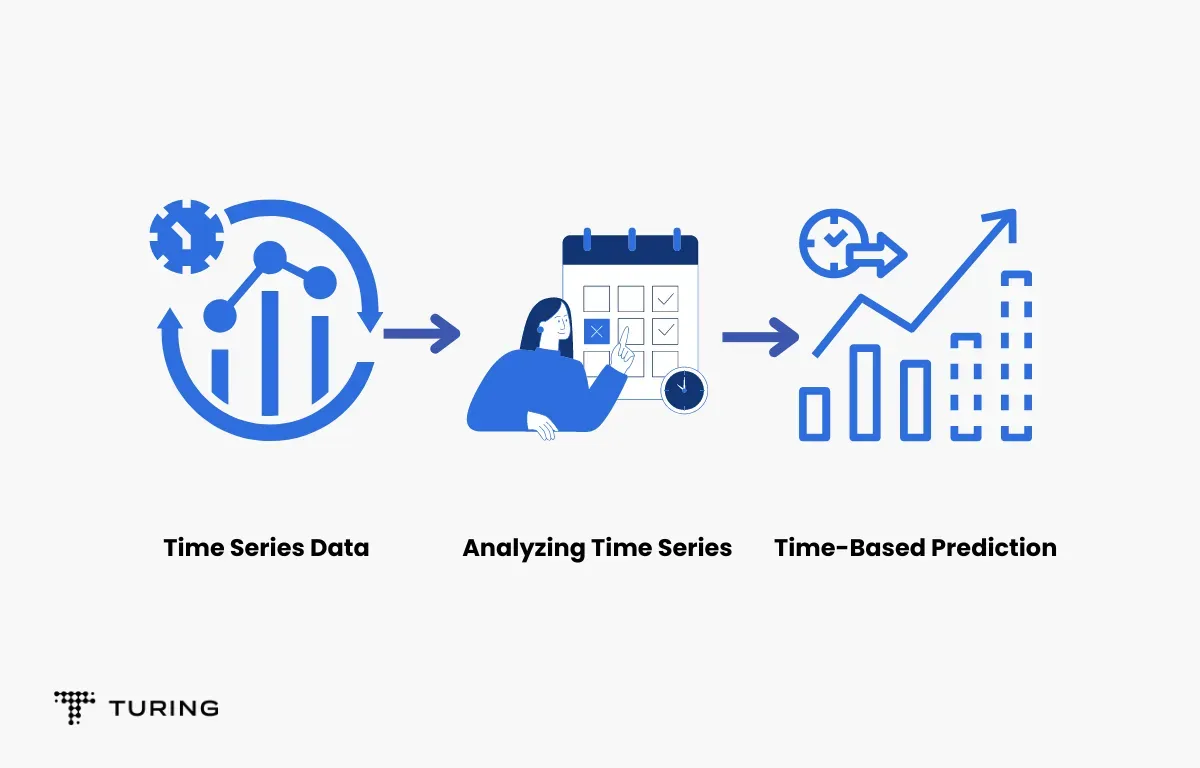Case in point: When you run a business for a while and have collected data for the past few months, you can predict which actions to take at what time. Sometimes, a business may be booming and you need to understand how to manage such volume. Other times, customers may come in search of a certain item, so you need to ensure you have it ready. With the help of time series analysis, you can predict all these things to help you differentiate and act accordingly.

## Components of time series analysis

The diagram below shows the components of time series analysis: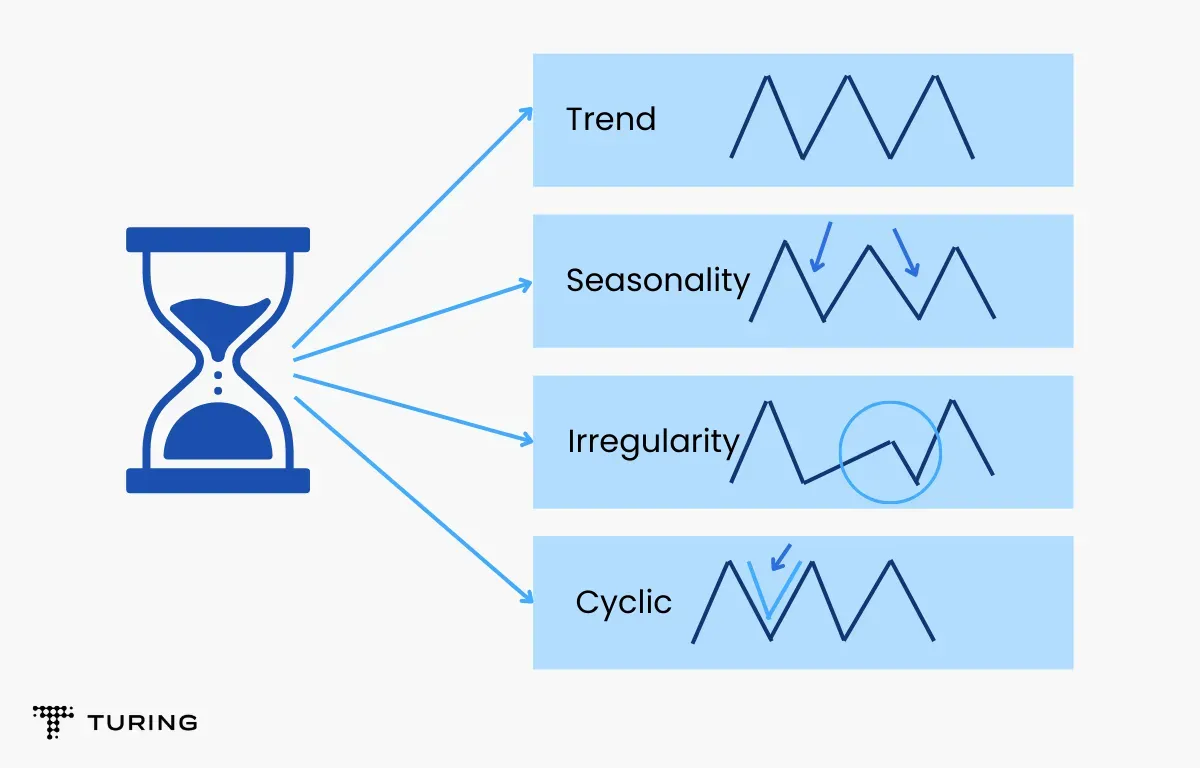1. Trend

Trend shows the variations in data by time or the frequency of the data. It lets you analyze the increase or decrease of data over time. The data can be stable or increase and decrease with population, market fluctuations, time, and productivity.

2. Seasonality

Seasonality is for the variations that occur at regular intervals. It can apply to festivals, seasons, and more. These will occur around the same time each year and their effect on data will be predictable.

3. Irregularity

There will not be any fluctuations in the time series data following seasons or trends. These variations in time will be random and will be unforeseen circumstances like natural disasters.

4. Cyclic

Oscillations in the time series that last more than a year are considered cyclic. They may or may not be periodic.

5. Stationary

A time series that has the same properties over time is stationary. These properties remain constant everywhere in the series. Your data should be constant in order to subject it to time-series analysis. A constant series has a constant variance, mean, and covariance.

## ARIMA model

An abbreviation of the autoregressive integrated moving average model, ARIMA is mainly used to predict the future values of a time series analysis using its previous values and forecasting errors.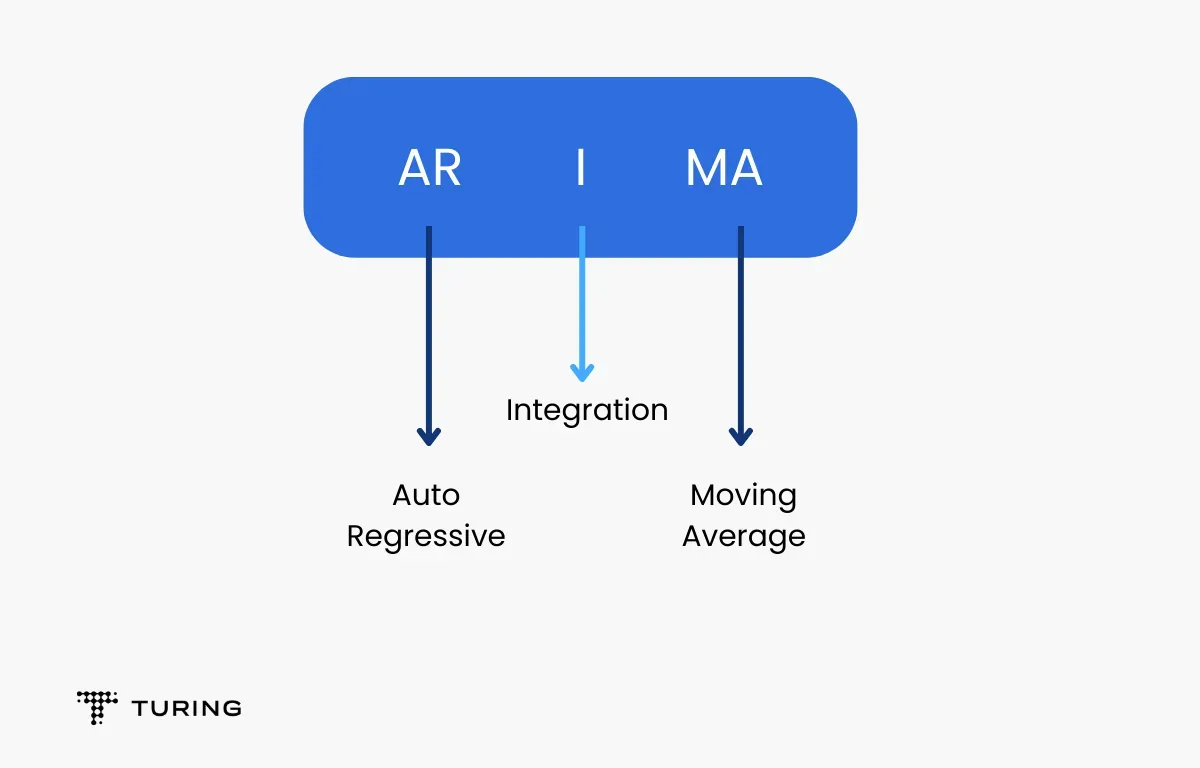### Autoregressive model

The autoregressive model predicts future values using past values where there are correlations between the past and future data. The formula for the autoregressive model is as below: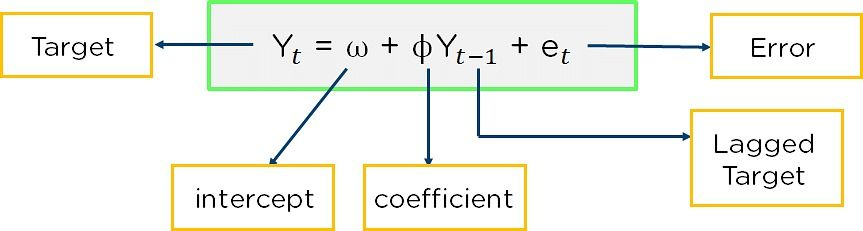Image source

The formula is the modified version of the slope formula where the target value is the sum of intercept, the product of a coefficient and the past output, and an error correction value.

### Moving average

The moving average is a statistical technique that takes the updated average of values to help cut down on noise. It takes the average of a specific time interval. You can get it by taking the different values of your data and finding their respective averages.

To do this, consider data points and take their average. Then, find the next average by removing the first value and including the next value in the series.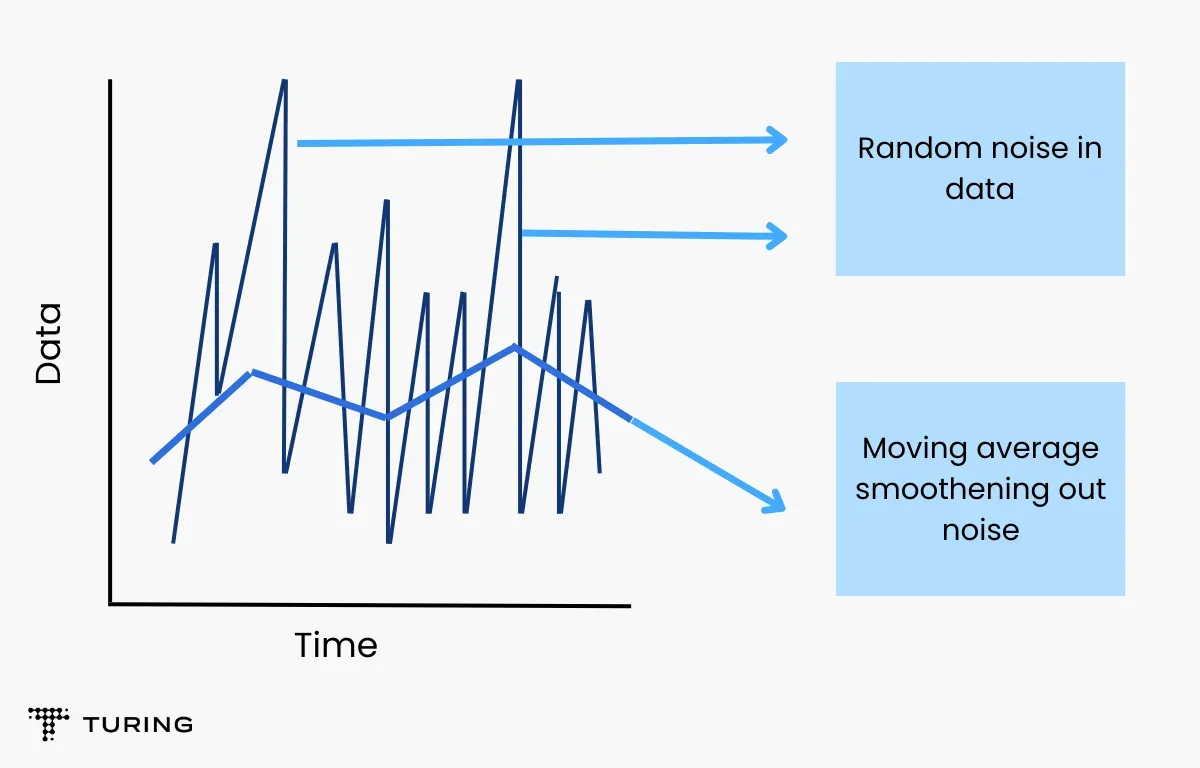### Integration

The integration model is the difference between the present and past observations and is used to make the time series constant.

Each of these values acts as a parameter for the ARIMA model. You can use them not only to represent the ARIMA model but also for other models and operators. The parameters are as follows:

• p: Past lagging values at each time point that is derived from the autoregressive model.
• q: Past lagging values for the error item that is derived from the moving average.
• d: Number of times data is different to make it constant and the times that integration is performed.

## Understanding ARIMA and ARMA

ARMA is a combination of autoregressive and moving average models for forecasting. It provides a weak constant process in terms of two polynomials, one for autoregression and the other for the moving average. ARMA is best used when you want to predict a constant series, whereas ARIMA supports constant as well as nonconstant series.

In ARIMA, autoregression uses past values to predict future ones. The moving average is used to analyze past errors and predict the future value.

Let’s understand the signature of ARIMA:

• p → log order → Number of observations
• d → differentiating degree → Number of times the raw observations are differenced
• q → order of MA → Size of the MA window

## Implementation steps for ARIMA

1. Below are the steps to follow to implement the ARIMA model:
2. Plot a time series format
3. Find the difference for making constant on mean by removing the trend
4. Make the variable constant by applying the log transformation
5. Note down the different log transformations for making constant on both mean and variance
6. Plot ACF and PACF and identify the potential autoregressive and moving average models
7. Discover the best fit for the ARIMA model
8. Forecast or predict the value using the best fit for the ARIMA model
9. Plott ACF and PACF for residuals of the ARIMA model, and ensure no information is left.

## Importing time series analysis in Python

The data for a time series is stored in .csv files or spreadsheet formats. It will contain two columns: the date and the measured value.

Let’s use the read_csv() in the Panda's package to read the time series dataset as a Pandas data frame. Adding the parse_dates=[‘date’] arguments will make the date column parse as a date field.

`from dateutil.parser import parse`

`import matplotlib as mpl`
`import matplotlib.pyplot as plt`

`import seaborn as sns`
`import numpy as np`
`import pandas as pd`

`plt.rcParams.update({‘figure.figsize’: (7,3), ‘figure.dpi’: 110})`

`df = pd.read_csv(‘https://company.com/dataman/datasets/master/s10.csv’, parse_dates=[‘date’])`
`df.head()`

You can also import it as a Pandas algorithm with the date as an index. You will need to specify the index.col argument in the pd.read_csv().

`ser = pd.read_csv(‘https://company.com/dataman/datasets/master/s10.csv’, parse_dates=[‘date’], index_col=’date’)`
`ser.head()`

In the above example, the column value is higher than the date which implies that it belongs to a series.

## What is panel data?

A panel data is a time-based dataset. The major difference between time-based datasets in addition to the time series is that it contains one or more related values that are measured for the same time intervals.

Typically, the present column in panel data will have explanatory variables that can help predict the Y by providing those columns for the future forecasting time.

## Moving average methodology

The most common technique used for time series analysis is the moving average methodology. This technique is clear with random short-term variations that are relatively linked with components of the time series. The rolling mean or moving average is calculated by taking an average of the data in the time series within k periods.

## Types of moving averages

Here are the three most important types of moving averages and their definitions:

1. Simple moving average (SMA): SMA is the unweighted mean of the previous points. The selection of sliding window datasets depends upon the leveling amount and the increase in the value that can improve the leveling at the expense of accuracy.

2. Exponential moving average (EMA): EMA is a technique mostly used to identify trends and filter out their noise. The weight for the recent data points is not historical. Compared to SMA, EMA responds faster to change and is more sensitive.

3. Cumulative moving average (CMA): CMA is the unweighted mean for the past values until the present time.

## Time series analysis in Machine Learning and Data Science

There are many model options available when dealing with time series analysis in machine learning and data science. Those with ARMA models have p, d, and q, where p is an auto-aggressive log, q is moving average lags, and d is a difference in order.

### Autocorrelation function (ACF)

ACF is used to indicate and see how similar a value will be within a given time series as well as its past value. It is also considered a measurement of the degree of similarity between different time series and the lagged version at different intervals observed. Python’s statsmodels library calculates the autocorrelation. It is used to identify a set of trends in the given dataset and influences it for the past observed values on the present observations.

### Partial autocorrelation function (PACF)

PACF is similar to ACF but can be challenging to understand. It shows the correlation of the sequence with some number of units per sequence in which only direct effects are shown. The ones with intermediary effects are removed from the time series.

### Relationship between ACF and PACF

The observations below highlight the example of temperature influences. The past temperature will influence the present one, but the significance of that will decrease and slightly increase the visualization with the temperature at regular time intervals.

### Interpreting ACF and PACF plots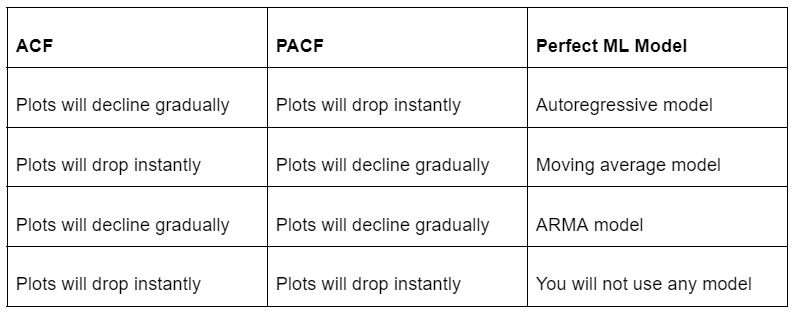It’s important to understand that both PACF and ACF require constant time series for analysis. An autoregressive model is a simple tool that predicts future performance based on previous performance. It is useful for forecasting correlations between values in a given time series and the values that are back and forth to it.

An autoregressive model uses a linear regression model that uses lagged values as its input. The linear regression model can easily be built with the help of the Scikit-learn library. This can be done by indicating the input for use. The statsmodels library is used to provide appropriate lag values and helps in training the model. It provides the AutoReg class to get the results using simple steps like:

• Creating a model with AutoReg() function
• Calling the Fit() function and training it on the dataset
• Returning an AutoRegResults Object
• Predicting by calling the Predict() function once it fits.

## Process flow

The use of time series analysis and forecasting in deep learning has increased over the years. It’s highly helpful for resolving problem statements that can’t be handled with ML techniques.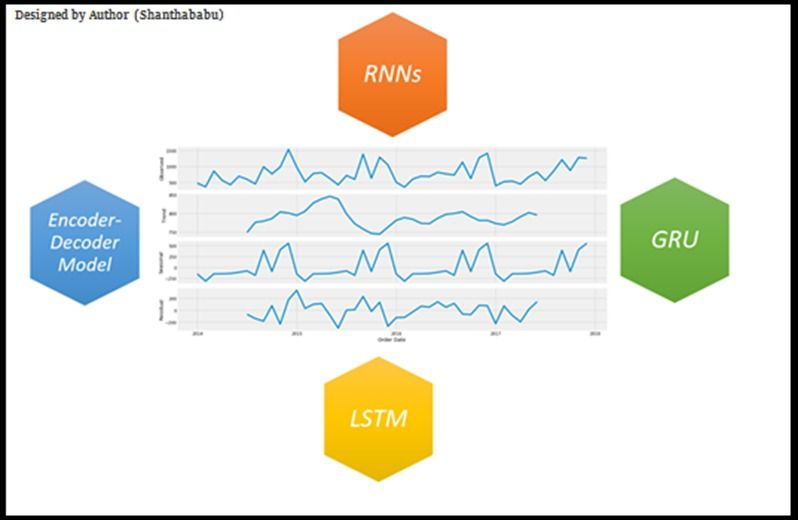Image source

Recurrent neural networks or RNNs are the most traditional architecture for time series forecasting based on the problems. RNNs are organized into successive layers and divided into input, hidden, and output. Each layer has equal weight and each neuron is assigned to a fixed time step. Remember that every one of them is fully connected to a hidden layer with the same steps. The hidden layers will be forwarded and will be time-dependent in direction.

The components of RNN are explained in detail below:

- Input: The function vector of x(t) is the input at the time t.
- Hidden: The function h(t) is the hidden layer at the time t. It is also a memory of an established network and calculates the current input and the previous hidden state.
- Output: The function vector y(t) is the output at the time t.
- Weights: The input vector connected to the hidden layer of neurons at the time t uses the weight U.

With time series analysis, you have a special feature that helps you remember every piece of information to assist in future predictions. It’s the ideal technique for creating complex patterns from required inputs. Though the computation cost is a little high, it delivers fast, efficient, and reliable predictions.### Press

What's up with Turing? Get the latest news about us here.### Blog